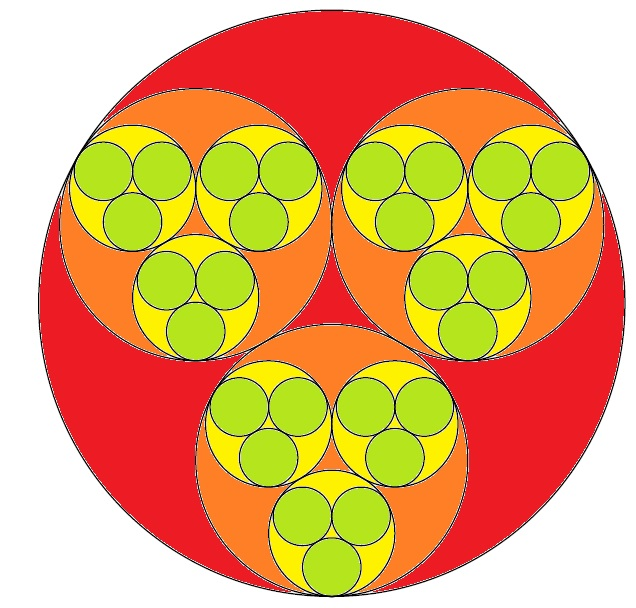# Circular Fractal Patters

Geometry Level 4Fractal Images are formed by geometrically repeating a pattern infinitely. Take for example the figure shown. Three smaller circles of equal radii are inscribed in a greater circle, where each smaller circle is tangent to the greater circle and the other two smaller circles. Then, each of the smaller circle circumscribes another set of even smaller circles of equal circles.

Let us say that the great circle drawn is a unit circle and we keep on drawing and drawing and drawing smaller circles following the pattern. What would the sum of the area of all the circles drawn in two decimal places?

×